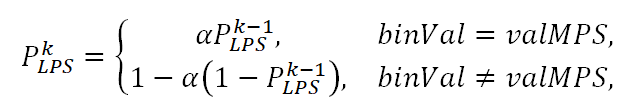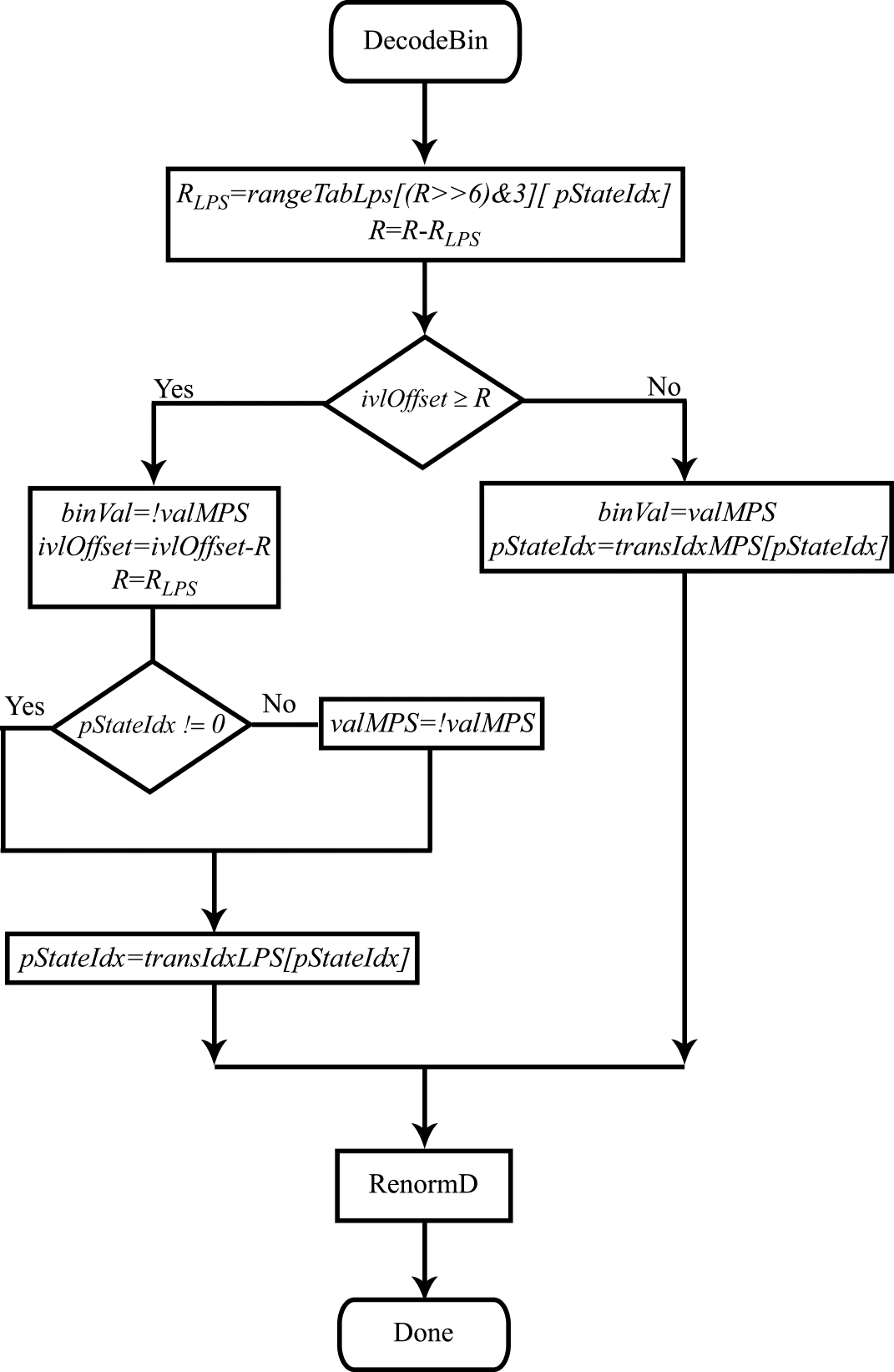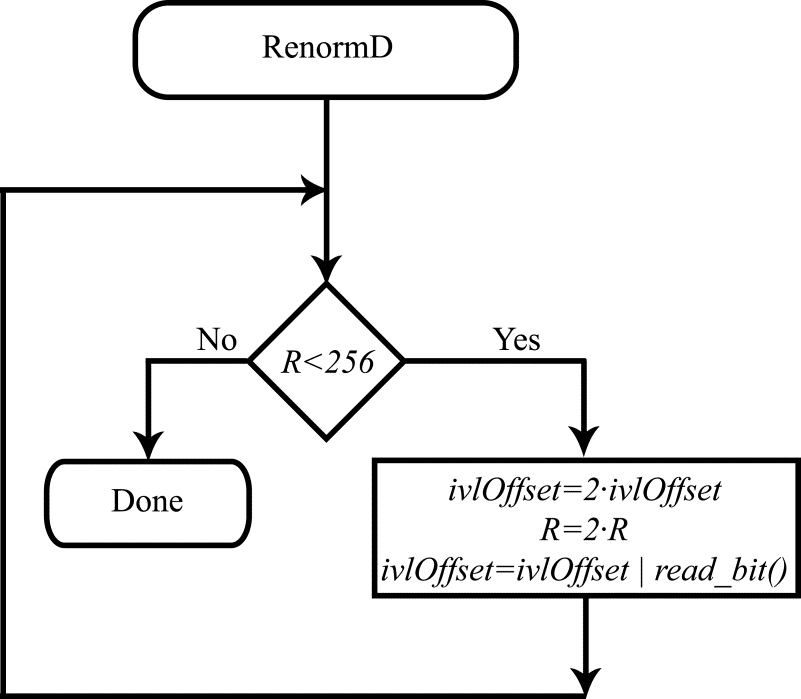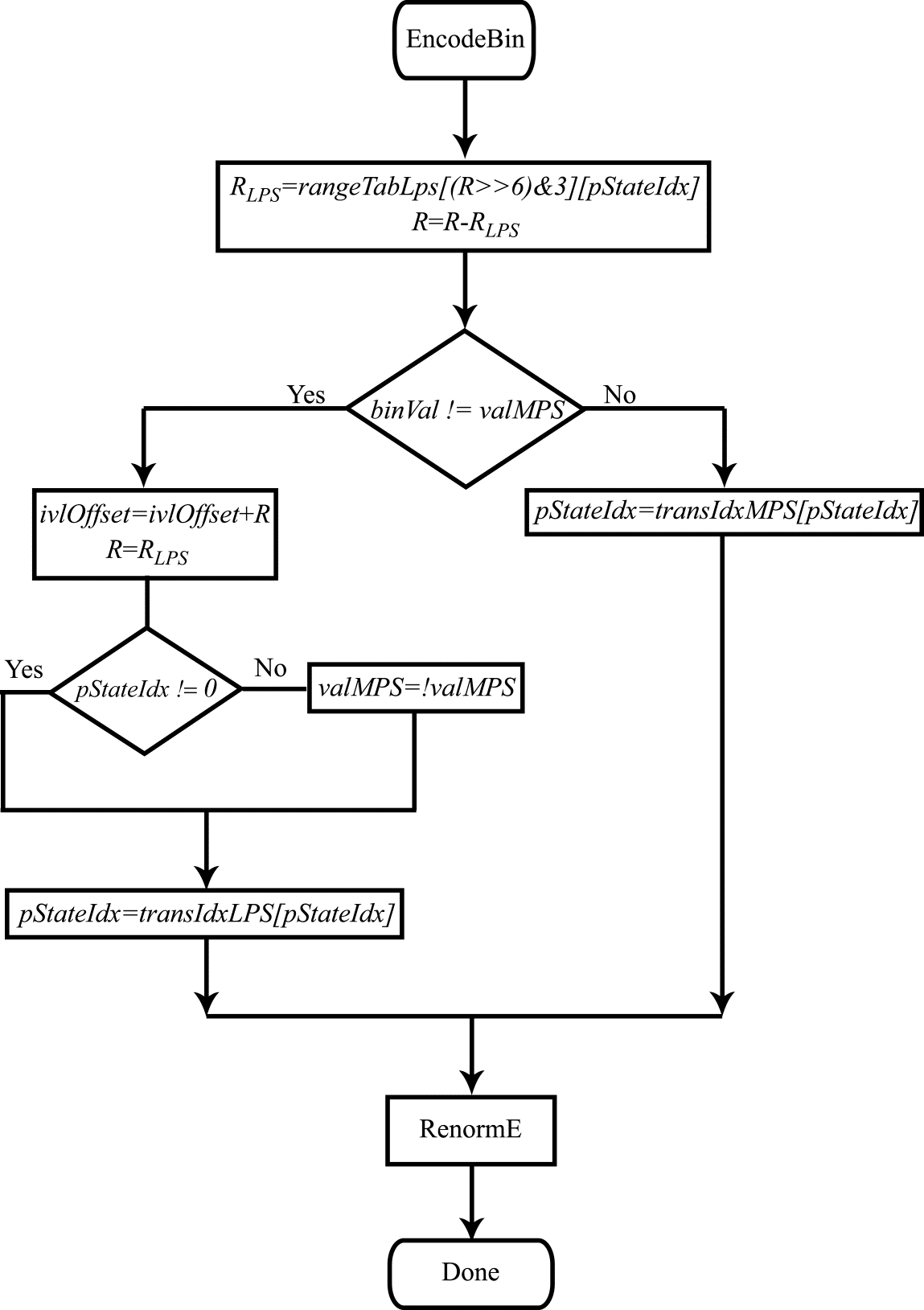# Context-adaptive binary arithmetic coding. Part 5

Context-adaptive binary arithmetic coding. Part 5

### CHAPTER 10### Using integer arithmetic for coding and the actual meaning of the “Context Adaptive” part in the term CABAC.

We have now given a brief analysis of the arithmetic encoding and decoding algorithms and discussed the procedures for transforming the values of syntax elements that describe the video frame content in an encoded stream into a binary bin stream, which is what is actually subjected to binary arithmetic coding. There are, however, some important things that we have not discussed yet. First, in the algorithms considered thus far, encoding and decoding have been done by splitting the current interval. The interval length is always less than 1, so the computations have to be performed using non-integer arithmetic. Second, encoding and decoding requires information on the probabilities of the symbols being encoded appearing, namely the probability of the least probable symbol $\displaystyle P_{LPS}$ occurring in the stream and the value of that symbol. Where do the encoder and decoder get this information? Finally, we still have not addressed the actual meaning of the “Context Adaptive” part in the term CABAC. Let us now deal with these remaining questions.

To answer the first question, the use of integer arithmetic for coding looks at first glance like an obvious possibility. One just needs to stretch the initial interval $\displaystyle [ 0,1)$ by an integer multiplier (e.g. 512), represent the probability $\displaystyle P_{LPS}$ as an integer divisor, and round off their quotient, after which all interval splitting operations can be performed as approximate computations using integer arithmetic with a specified resolution. The only unknown here is how much efficiency (i.e. compression ratio) will be lost due to the approximation. As early as the beginning of the 1990s, a number of theorems were published and proved that made it possible to estimate the loss of compression ratio (see, for example, P. G. Howard, J. S. Vitter Analysis of Arithmetic Coding for Data Compression, Information Processing and Management 28 (1992), 749 – 763). These losses resulting from the approximate nature of the computations turned out to be minimal. Thus, it was proven that efficient implementation of binary arithmetic coding algorithms based on integer arithmetic is possible.

The developers of CABAC went even further in that direction. They suggested coarsening the values of the current interval length $\displaystyle R$ and probability $\displaystyle P_{LPS}$ to such an extent as to be able to compute all possible values of the product $\displaystyle R_{LPS} =R\cdotp P_{LPS}\\$ in advance. The resulting proposed CABAC algorithm replaces the computationally intensive multiplication operation with a lookup from a table populated with pre-computed multiplication results (in the standard, see Table 9-40 – Specification of rangeTabLps depending on the values of pStateIdx and qRangeIdx). Let us consider this in more detail.

The current interval length $\displaystyle R$ is represented in CABAC by a 9-bit variable called ivlCurrRange. It is assigned an initial value of 510 at the initialization stage of the encoding/decoding process. The values of this variable after encoding/decoding each bin and performing the renormalization procedure always fall in the range of 257 to 511. Before performing the multiplication $\displaystyle R_{LPS} =R\cdotp P_{LPS}\\$, the value $\displaystyle R$ is quantized, i.e. divided by 64 (the quantizing is implemented as a right-shift by 6 bits). Since the 8th bit of this variable (with 0-based numbering, here 0 to 8) is always 1, the four possible quantized values of $\displaystyle R$ represent the 6th and 7th bits of ivlCurrRange. It is the value of $\displaystyle ( ivlCurrRange\gg 6) \&3$ that is used as one of the addresses to look up pre-computed values of the multiplication result from the two-dimensional rangeTabLps table. The second index ranging from 0 to 63 represents the 64 possible values of $\displaystyle P_{LPS}$. Here we are approaching the answer to the second question: How is the probability $\displaystyle P_{LPS}$ computed during encoding/decoding?

The probability value $\displaystyle P_{LPS}$ is updated recursively each time a new value of the bin to be encoded/decoded ($\displaystyle binVal$) is obtained. At the $\displaystyle k$th step (that is, during the encoding or decoding of the $\displaystyle k$th bin), the new value of $\displaystyle P^{k}_{LPS}$ is computed as follows:where $\displaystyle α=\left(\frac{0,01875}{0,5}\right)^{\frac{1}{63}}$, and $\displaystyle valMPS$ is the value of the most probable symbol (0 or 1). As was already mentioned, $\displaystyle P_{LPS}$ takes 64 possible values that are indexed by the 6-bit $\displaystyle pStateIdx$ variable in CABAC. Updating the probability value amounts to updating the index $\displaystyle pStateIdx$ and is carried out by looking up values from pre-computed tables (see Table 9-41, State transition table, in the standard). When $\displaystyle pStateIdx$ takes a value of 0, $\displaystyle valMPS$ changes its value to the opposite. The value of $\displaystyle pStateIdx$ is also used as the second index when looking up values from the rangeTabLps table, i.e. when determining the value of the product $\displaystyle R_{LPS} =R\cdotp P_{LPS}\\$.

The flow diagram for the decoding algorithm modified to use integer arithmetic is shown in Figure 1.Figure 1. Flow diagram for the bin decoding procedure.

The flow diagram for the decoder renormalization procedure is shown in Fig. 2.Figure 2. Flow diagram for the decoder renormalization procedure.

The flow diagrams for the encoding and encoder renormalization algorithms are shown in Figs. 3 & 4.Figure 3. Flow diagram for the coding procedure.Figure 4. Flow diagram for the encoder renormalization procedure.

Finally, let us make sense of the “Context Adaptive” part in the name of the CABAC coding procedure. This is also pretty simple. The values of all bins subjected to arithmetic coding are obtained by binarizing the values of various syntax elements. The binarization algorithms are different for different elements. As a result, the bins that represent the values of different syntax elements have different probabilities for the zero and one values. Moreover, the probability $\displaystyle P_{LPS}$ typically depends on the ordinal number of the bin in the binarized representation of the syntax element value. This probability, as well as the $\displaystyle valMPS$ value, can vary as a function of the values of this syntax element in the adjacent blocks of the video frame. In this situation, it makes good sense to evaluate the probability $\displaystyle P_{LPS}$ and the $\displaystyle valMPS$ separately for each group of bins that have approximately the same statistics. This is what the CABAC encoding/decoding procedure does. As was mentioned above, the discrete values of the probability $\displaystyle P_{LPS}$ are defined in CABAC using the 7-bit index $\displaystyle pStateIdx$. This index together with the 1-bit $\displaystyle valMPS$ value fully defines the bin statistics. The values of $\displaystyle pStateIdx$ and $\displaystyle valMPS$ are updated separately for each individual group of same-type bins. For all such bins, these two values (combined into one 8-bit value) are stored in a special table called ctxTable. The elements of this table are referred to in the standard as the context. The context modeling procedure, which is part of the CABAC encoding/decoding algorithms, consists of determining the index $\displaystyle ctxIdx$ in order to get the values of $\displaystyle pStateIdx$ and $\displaystyle valMPS$ from this table. The index value $\displaystyle ctxIdx$ is determined by the type of the syntax element to whose binarized representation the current bin belongs, the number of the current bin in this binarized representation, and possibly the values of this syntax element in the adjacent blocks of the video frame being coded. The context is thus selected adaptively depending on the group of statistically similar bins that the current segment belongs to. Upon the completion of the encoding/decoding procedures during which $\displaystyle pStateIdx$ and $\displaystyle valMPS$ are updated, the updated values are stored in ctxTable at the corresponding address, $\displaystyle ctxIdx$.

With this we conclude the discussion of the CABAC encoding/decoding algorithms. There are a quite a few additional aspects not addressed here that could become subjects of further articles. On the other hand, we have successfully analyzed the main ideas that underlie the implementation of CABAC and worked our way up from a simple example of arithmetic coding to flow diagrams that describe the encoding/decoding algorithms in the HEVC standard.

March 23, 2020

Chapter 1. Video encoding in simple terms

Chapter 2. Inter-frame prediction (Inter) in HEVC

Chapter 3. Spatial (Intra) prediction in HEVC

Chapter 4. Motion compensation in HEVC

Chapter 5. Post-processing in HEVC

Chapter 6. Context-adaptive binary arithmetic coding. Part 1

Chapter 7. Context-adaptive binary arithmetic coding. Part 2

Chapter 8. Context-adaptive binary arithmetic coding. Part 3

Chapter 9. Context-adaptive binary arithmetic coding. Part 4